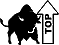If you need help in beginning algebra, you have come to the right place.  Note that you do not have to be a student at WTAMU to use any of these online tutorials.  They were created as a service to anyone who needs help in these areas of math.

If this is your first time using this Beginning Algebra Online Tutorial please read the Guide to the WTAMU Beginning Algebra Online Tutorial Website to learn how our tutorials are set up and the disclaimer.  Come back to this page to make your tutorial selection.

Please click on the name of the tutorial of your choice:

Tutorial 1:  How to Succeed in a Math Class

Tutorial 2:  Symbols and Sets of Numbers

Tutorial 3:  Fractions

Tutorial 5: Adding Real Numbers

Tutorial 6: Subtracting Real Numbers

Tutorial 7: Multiplying and Dividing Real Numbers

Tutorial 8: Properties of Real Numbers

Tutorial 9: Reading Graphs

Tutorial 10: Practice Test on Tutorials 2 - 9

Tutorial 11: Simplifying Algebraic Expressions

Tutorial 12: The Addition Property of Equality

Tutorial 13: The Multiplication Property of Equality

Tutorial 15: Introduction to Problem Solving

Tutorial 16: Percent and Problem Solving

Tutorial 17: Further Problem Solving

Tutorial 18: Solving Linear Inequalities

Tutorial 19: Practice Test on Tutorials 11 - 18

Tutorial 20: The Rectangular Coordinate System

Tutorial 21: Graphing Linear Equations

Tutorial 22: Intercepts

Tutorial 23: Slope

Tutorial 24: Graphing Linear Inequalities

Tutorial 25: Practice Test on Tutorials 20 - 24

Tutorial 26: Exponents

Tutorial 27: Adding and Subtracting Polynomials

Tutorial 28: Multiplying Polynomials

Tutorial 29: Negative Exponents and Scientific Notation

Tutorial 30: Division of Polynomials

Tutorial 31: Practice Test on Tutorials 26 - 30

Tutorial 32: Formulas

Tutorial 33: Basic Geometry

Tutorial 34: Central Tendencies

Tutorial 35: Reasoning Skills

Tutorial 36: Practice Test on Tutorials 32 - 35[Copyright]  [Fair Use]  [Intellectual Property]  [Resource Guide]